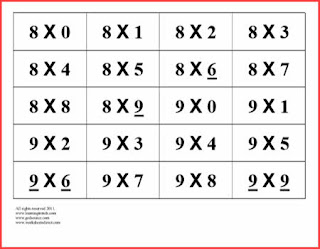## Friday, December 07, 2018

### GED Math and Basic Math Facts

Learning the basic facts of addition, subtraction, multiplication, and division are an important part of any math foundation. I am a firm believer that every student, whether a child or adult, needs to know their basic facts, without using their fingers, a chart, or a calculator. Having a solid foundation and a firm understanding in this area will better equip a student for learning fractions, decimals, percent, algebra, geometry, etc. If a student does not knows their math facts, they will struggle in math all the way through school. When learning or reviewing these facts, use a variety of methods and resources.For additional math resources worksheetsheetsdirect

This is especially important for students who are studying to take their GED math test. The stronger a learner's basic knowledge of math skills, including basic math facts, the quicker a student can complete the math problems.  There are some great resources on the internet for downloading math flashcards or interactive activities for reinforcing your student's basic math facts.